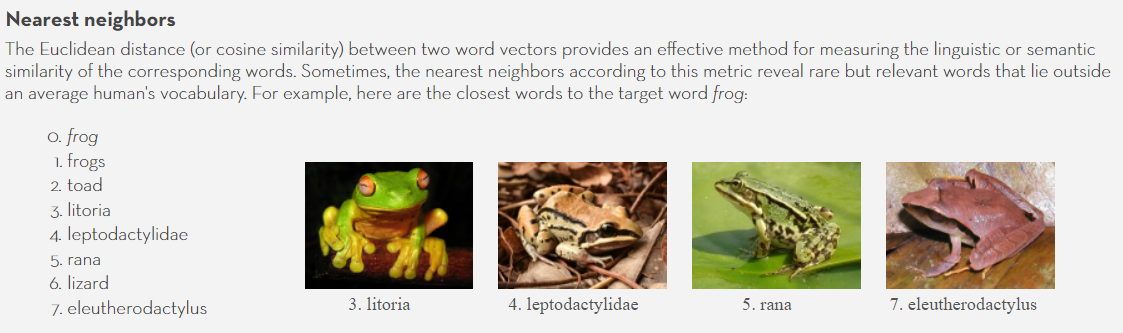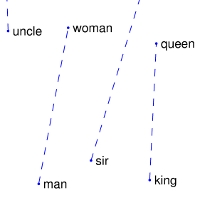# Word Similarities

One of the more popular characteristics of Word Embeddings is that it affords a way to look at the similarity between words.

Canonically, the GloVe embedding boasts the ability to serve up words in similar feature space and demonstrate that they have similar meaning.

from IPython.display import Image

Image('images/glove_nearest.PNG')The above merely considers the straight-line distance between two points, but cosine similarity has been a shown to be a more effective similarity measure when working with text data. Mathematically, we can represent this with

$sim(u, v) = \frac{u^Tv}{\Vert{u}\Vert_2 \Vert{v}\Vert_2 }$

Or we could import it for simple use from sklearn

from sklearn.metrics.pairwise import cosine_similarity

### Composability

Finally, a characteristic of a good Word Embedding matrix is the ability to do “word math” with words in your vocabulary. One of the more canonical examples of this is

man - woman ~ king - queen


This is because, all things equal, the only difference between each pair of words should be on one “gender” axis.

Image('images/man_woman_small.jpg')As an extension of this, we can create a fun word analogy calculator (borrowed from Andrew Ng’s 5th Deep Learning Coursera course) that gets the cosine similarity between two words, then finds the partner word for a third input that closest-resembles the relationship of the first two.

def complete_analogy(word_a, word_b, word_c, word_to_vec_map):
"""
Performs the word analogy task as explained above: a is to b as c is to ____.

Arguments:
word_a -- a word, string
word_b -- a word, string
word_c -- a word, string
word_to_vec_map -- dictionary that maps words to their corresponding vectors.

Returns:
best_word --  the word such that v_b - v_a is close to v_best_word - v_c, as measured by cosine similarity
"""

word_a, word_b, word_c = word_a.lower(), word_b.lower(), word_c.lower()

e_a = word_to_vec_map[word_a]
e_b = word_to_vec_map[word_b]
e_c = word_to_vec_map[word_c]

words = word_to_vec_map.keys()
max_cosine_sim = -100
best_word = None

vec = e_b - e_a

for w in words:
if w in [word_a, word_b, word_c] :
continue

attempt = word_to_vec_map[w] - e_c
cosine_sim = cosine_similarity(vec, attempt)

if cosine_sim > max_cosine_sim:
max_cosine_sim = cosine_sim
best_word = w

return best_word